Test: Fluid Mechanics Level - 4

# Test: Fluid Mechanics Level - 4 - Mechanical Engineering

Test Description

## 25 Questions MCQ Test - Test: Fluid Mechanics Level - 4

Test: Fluid Mechanics Level - 4 for Mechanical Engineering 2023 is part of Mechanical Engineering preparation. The Test: Fluid Mechanics Level - 4 questions and answers have been prepared according to the Mechanical Engineering exam syllabus.The Test: Fluid Mechanics Level - 4 MCQs are made for Mechanical Engineering 2023 Exam. Find important definitions, questions, notes, meanings, examples, exercises, MCQs and online tests for Test: Fluid Mechanics Level - 4 below.
Solutions of Test: Fluid Mechanics Level - 4 questions in English are available as part of our course for Mechanical Engineering & Test: Fluid Mechanics Level - 4 solutions in Hindi for Mechanical Engineering course. Download more important topics, notes, lectures and mock test series for Mechanical Engineering Exam by signing up for free. Attempt Test: Fluid Mechanics Level - 4 | 25 questions in 50 minutes | Mock test for Mechanical Engineering preparation | Free important questions MCQ to study for Mechanical Engineering Exam | Download free PDF with solutions
 1 Crore+ students have signed up on EduRev. Have you?
Test: Fluid Mechanics Level - 4 - Question 1

### When a block of ice floating on water in a container melts, the level of water in the container

Test: Fluid Mechanics Level - 4 - Question 2

### The body whose surface does not coincide with the streamline when places in a flow is called as

Test: Fluid Mechanics Level - 4 - Question 3

### A large metacentric height in a vessel

Test: Fluid Mechanics Level - 4 - Question 4

The time of oscillation of a floating body is given by Where
K = Radius of Gyration of the floating body about its centre of gravity
h = Metacentric height of the floating body

Test: Fluid Mechanics Level - 4 - Question 5

A cylindrical body of cross-sectional area A height H and densityrs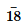is immersed to a depth h in a liquid of density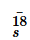r and tied down to bottom with a string then the tension in the string is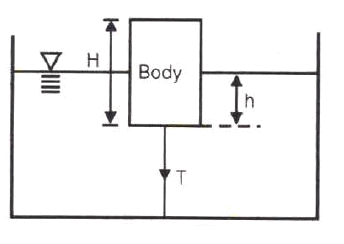Test: Fluid Mechanics Level - 4 - Question 6

There is no geometrical distinction between the streamline, pathline and streak line in case of

Test: Fluid Mechanics Level - 4 - Question 7

Stream line, path line and streak line are identical when the

Test: Fluid Mechanics Level - 4 - Question 8

Match List I with List II and select the correct answer using the codes given below the lists: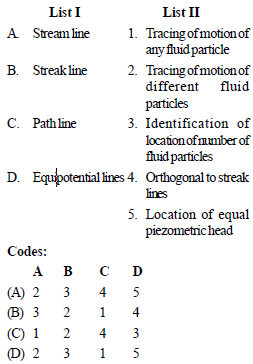Test: Fluid Mechanics Level - 4 - Question 9

The continuity equation in differential form is

Test: Fluid Mechanics Level - 4 - Question 10

Under which of the following conditions will the equation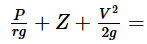constant, will be valid in the whole flow field ?

1. Flow is rotational
2. Flow is irrotational
3. Flow is incompressible
5. Flow is laminar

Test: Fluid Mechanics Level - 4 - Question 11

Venturimeter consists of following parts :
1. Diverging part
2. Throat
3. Main inlet pipe
4. Short converging part
Select the correct sequence of parts

Test: Fluid Mechanics Level - 4 - Question 12

The range for coefficient of discharge (Cd) for a venturimeter is

Test: Fluid Mechanics Level - 4 - Question 13

In the siphon in figure below assuming ideal flow, the pressure PB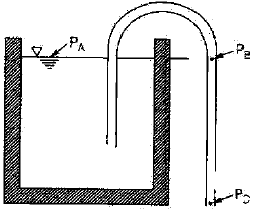Test: Fluid Mechanics Level - 4 - Question 14

The flow of fluid through a pipe is laminar when

Test: Fluid Mechanics Level - 4 - Question 15

An oil of kinematic viscosity 0.5 stokes flows through a pipe of 4 cm diameter. The flow is critical at a velocity of about

Test: Fluid Mechanics Level - 4 - Question 16

In the Navier-Stokes equations, the forces considered are

Test: Fluid Mechanics Level - 4 - Question 17

Navier-Stokes equation represents the conservation of

Detailed Solution for Test: Fluid Mechanics Level - 4 - Question 17

The Navier-Stokes equations govern the motion of fluids and can be seen as Newton's second law of motion for fluids. ... The Navier-Stokes equations represent the conservation of momentum, while the continuity equation represents the conservation of mass.

Test: Fluid Mechanics Level - 4 - Question 18

For laminar flow between two fixed parallel plates, the flow velocity

Test: Fluid Mechanics Level - 4 - Question 19

Capillary tube viscometers used for measurement of viscosity are based on

Test: Fluid Mechanics Level - 4 - Question 20

An incompressible fluid (kinematic viscosity, 7.4 × 10–7 m2/s, specific gravity, 8.88) is held between two parallel plates. If the top plate is moved with a velocity of 0.5 m/s while the bottom one is held stationary, the fluid attains a linear velocity profile in the gap of 0.5 mm between these plates; the shear stress in Pascals on the surface of top plate is

Test: Fluid Mechanics Level - 4 - Question 21

Pressure drop in a 100 mm diameter horizontal pipe is 50 kPa over a length of 10 m. The shear stress at the pipe wall is

Test: Fluid Mechanics Level - 4 - Question 22

The pressure drop for a relatively low Reynolds number flow in a 600 mm, 30 m long pipe line is 70 kPa. What is the wall shear stress

Test: Fluid Mechanics Level - 4 - Question 23

When the hydrodynamic boundary layer thickness (δ), which is the distance from the surface at which the local velocity (u) reaches 99% of the free-stream velocity, then

Test: Fluid Mechanics Level - 4 - Question 24

Shear velocity is

Test: Fluid Mechanics Level - 4 - Question 25

The head loss in a sudden expansion from 5 cm diameter to 10 cm diameter pipe, in terms of velocity V1 in the 5 cm pipe

Information about Test: Fluid Mechanics Level - 4 Page
In this test you can find the Exam questions for Test: Fluid Mechanics Level - 4 solved & explained in the simplest way possible. Besides giving Questions and answers for Test: Fluid Mechanics Level - 4, EduRev gives you an ample number of Online tests for practice(Scan QR code)